Before we begin, make sure to download the left lookup example file below:

VLOOKUP is very useful for finding and retrieving related information in a table (or dataset). This function isn’t perfect though. It suffers from certain limitations.

One of the limitations VLOOKUP impose is the fact that the lookup column must be the first column in the range. That means you cannot perform VLOOKUP to the left of the key field.

The very well-known technique to avoid this particular VLOOKUP limitation is to use other functions (the MATCH and INDEX functions) instead of the VLOOKUP function. In this tutorial, we’ll look at these functions one after the other and how to use them to perform a left lookup.

Note: Before I proceed, let me mention that there’s no ideal way VLOOKUP can look to the left of the key field (not even in the 2019 version of Excel). Therefore, to look to the left, you will have to use the technique here. However, if you must use the VLOOKUP function, the obvious solution is to switch columns in your data so that the lookup column will come first before the index_num column.

## Understanding the MATCH function

The MATCH() function is used to look for a value in a range and then retrieve the position of the cell that matches the specified value. The MATCH() function depends on other functions to be useful. It doesn’t do much on its own.

This function goes with three arguments outlined below:

### MATCH Function Syntax:

MATCH(lookup_value, lookup_array, [match_type])

• lookup_value: This represents the value you are looking for. This could either be text or numbers.
• lookup_array: This is the range you are searching through. The range must be one-dimensional. This means that you can only search through one column or one row (like A1:A10 or A1:G1), but you can’t search a grid of cells (like A1:G10 which include several rows and columns).
• match_type: This argument is used to specify how the match is determined. A match_type of 0 (the number zero) will retrieve the position of the first item that matches exactly. In most cases, the 0 match_type is used so I recommend you to always stick to it. A match_type of 1 will retrieve the position of the largest value that’s equal to or less than the searched value. A match_type of -1 gives you the position of the smallest value that’s equal to or greater than the searched value.

Note: The match_type of 1 and -1 are rarely used in the real world. So always stick to using a match type of 0. Also, if you fail to specify a match type, Excel will automatically assign 1 as your match type which may not be good for you. Consider this as a warning.

## Example of MATCH() function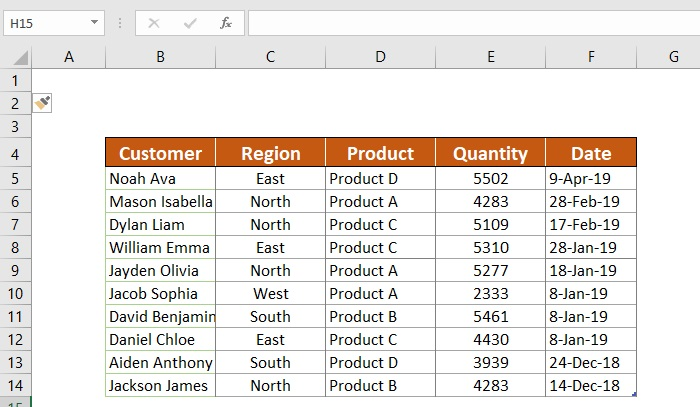Above is a table that contains customer order information. And there are about 10 records in this table.

To illustrate the MATCH() function, let’s write a formula to retrieve the position of a customer named Jacob Sophia in the customer list.The MATCH() formula in cell D2 says the name “Jacob Sophia” is in the 6th location in the list. However, if there’s no match, the function will return #N/A which means that what you are looking for is not in that column.

See screenshot: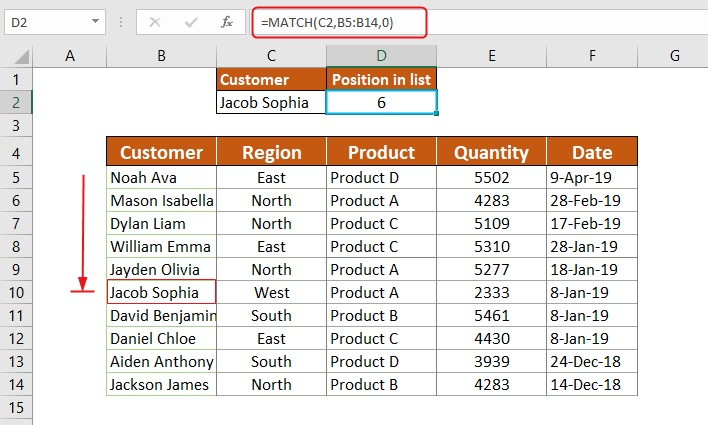To grasp how this formula works, you’ll have to try it yourself. Also, check this step by step guide on how to use the MATCH function.

## Understanding the INDEX function

As you can see, the MATCH() function only does half of the work of VLOOKUP by returning only the position of the search term. It can’t even retrieve the actual content of the cell.

It is clear that the MATCH() function isn’t so useful on its own. It becomes useful only when you combine it with another function (like the INDEX function)

The INDEX() function retrieves a value from a range of cells, using the index number you specify.

This function has the following arguments:

### INDEX Function Syntax:

INDEX(array, row_num, [column_num])

• array: This represents a range of cells.
• row_num: This represents a row number in a range.
• column_num: This represents a column number in a range.

INDEX() function exampleUsing the table above, lets illustrate how the index function works.

Assuming the above table is sorted in a way that, the first name in the list is our first customer, the second name as our second customer, then in that order up to the end.

Using the index function, we’ll find and retrieve the product our 5th customer bought. So, consider the following formula:

=INDEX(B5:F14,5,3)This formula gives you the content of the cell in the 5th row of the 3rd column, which is cell D9.

But how?

The first argument (B5:F14) specifies the range of cells we are interested in. You can also use a named range for this argument, like CustomerTable.

The second argument also finds the row we want, and that is the 5th row.

Finally, the third argument represents the index number of the product column which is 3.

Altogether, the formula retrieves the 5th value in the 3rd column of the table.

See screenshot:## “Left lookup” using INDEX-MATCH

These two functions (the MATCH and INDEX functions) are often used in each other’s company to perform lookups.

On their own, they are almost useless. But when they are used together, they have the ability to overcome the various VLOOKUP limitations.

## Example of Left lookup

Consider the following example:

In the example below, using the Customer column, the formula retrieves information from the Date column which is found at the left side of the lookup column (Customer). This is a clear example of VLOOKUP to the left (or left lookup), something the VLOOKUP() function cannot handle.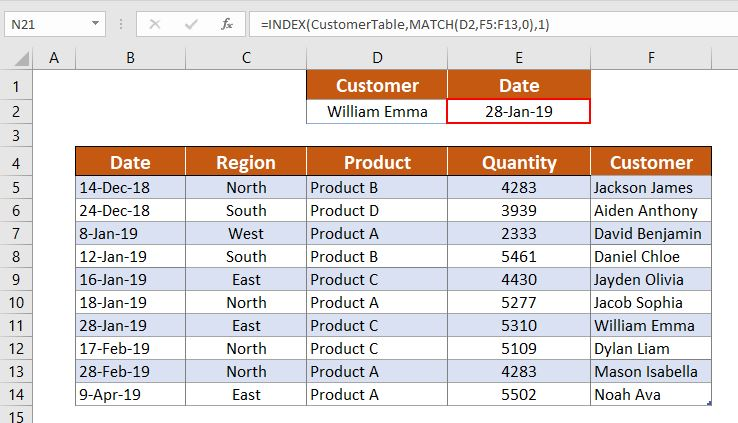This worksheet lets you enter a customer by name, and then retrieve the corresponding date on which the customer made a purchase. Since the Date column is to the left of the Customer column (or lookup column), VLOOKUP can’t perform this task. The solution is to use the MATCH INDEX technique.

## Left lookup breakdown

Considering the above example, to lookup to the left, first find the position of the product you are looking for using the MATCH() function:

=MATCH(D2,F5:F14,0)

If you run this formula alone, you’ll get a value of 7 because the search term (William Emma) is located in the 7th row of the table.

Now, you can get the position of any lookup value, thanks to the MATCH() function.

With this position details you can also retrieve any corresponding cell content by employing the INDEX() function.

All you have to do is use the MATCH() function as the second argument (row_num) in the INDEX() function, then specify the column from which you want to retrieve the corresponding value. And bam! Your formula can now look to the left.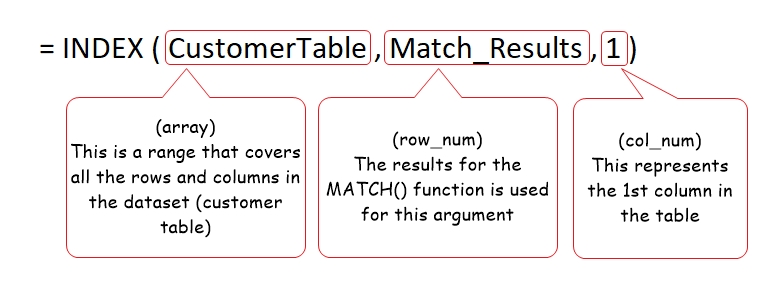Here’s how you’d merge these functions into a single formula:

=INDEX(CustomerTable,MATCH(D2,F5:F14,0),1)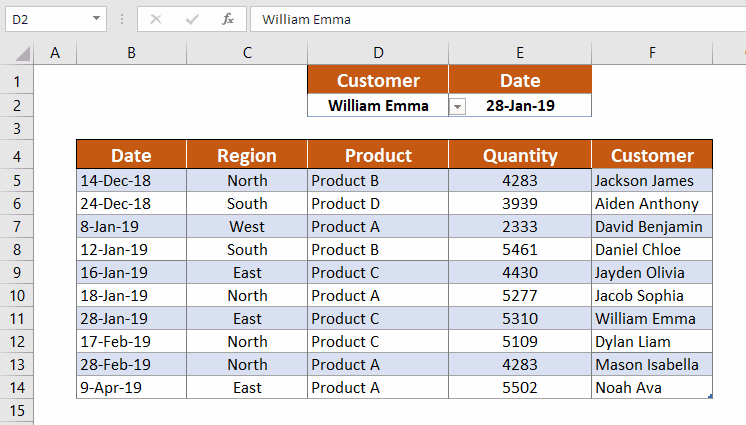These two functions, when combined together, can be a very powerful tool for advanced Excel users. Apart from using them for the left lookup (or VLOOKUP to the left), you can also use them to perform a double lookup.